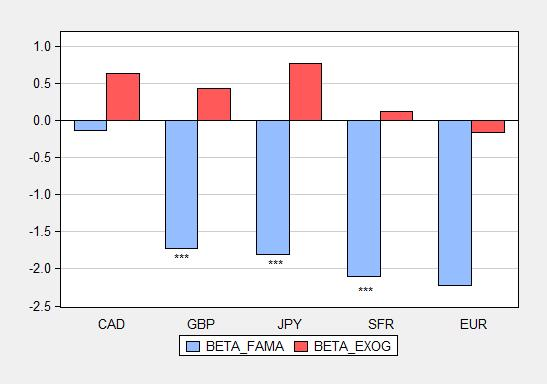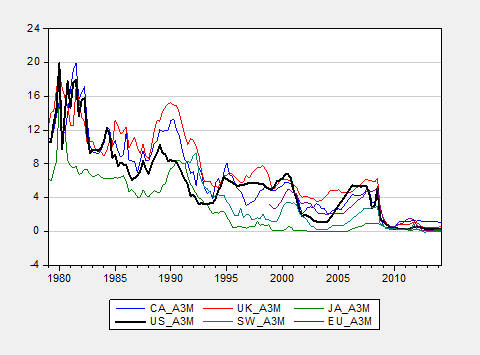# Interest Rate Parity and Exogeneity

One of the enduring puzzles of international finance is the fact that the joint hypothesis of uncovered interest parity and rational expectations is consistently rejected, as evidenced by the coefficient estimates in the Fama regression.

The typical approach is to estimate a regression of the ex post change (in logs) of the exchange rate on the interest differential (this is an approximation).

(1)     Δst+1 = β0 + β1 (it – it*) + εt+1

A commonplace finding is that estimates of β1 are negative and statistically significantly different from unity, which is the posited value under the null hypothesis. Estimates for five bilateral exchange rates (against the US dollar, 1979-2014Q2) are shown as blue bars in Figure 1.Figure 1: β OLS estimates from equation (1), quarterly data, 1979-2014Q2 period. *** denotes statistically significantly different from unity at 1% level.

One requirement for ordinary least squares (OLS) to yield unbiased estimates is the right hand side variable is uncorrelated with the error term. If the interest rate differential is endogenous (say monetary policy adjusts the interest rate in response to factors impounded in the error term), then estimates could be biased. In Chinn and Meredith (2004), we explicitly model interest rate policy as a function of output and inflation gaps, and inflation and output are a function of exchange rate depreciation; hence in that framework, the right hand side variable is by construction correlated with the error term. A similar approach using a DSGE is undertaken by Chinn and Zhang (2015).

My view is that US monetary policy is less subject to this critique, given the more closed nature of the American economy. One could proceed, using instrumental variables (all one needs are valid instruments). Another approach is to move the endogenous variable to the left hand side, imposing a unit coefficient.

(2)     Δst+1 – it = β’0 – β1‘ it* + ε’t+1

The β1‘ estimates are shown as red bars in Figure 1. Notice that while in a couple cases the β’s appear numerically far away from unity, in no case are the estimates statistically distinguishable from unity.

Is this necessarily the explanation for the biased estimates? It does seem plausible that US interest rates are more exogenous than foreign. Figure 2 plots the 3 month (CD) series used.Figure 2: Three month offshore interest rates, end-of-period, %, for US (bold black), Canada (blue), UK (red), Japan (green), Switzerland (chartreuse), and euro (purple).

There might be other explanations, reviewed in for instance Chinn (2006), including more econometric ones (Chinn and Meredith (2005)). An alternative interpretation is that imposing the same β1 on US and foreign interest rates induces correlation between the right hand side variable and the error term.

More on updated results on the Fama regression in this post. Results using survey data as opposed to assuming rational (unbiased) expectations, in this paper.

## 4 thoughts on “Interest Rate Parity and Exogeneity”

There may be some systematic risk.

For example, it’s much easier to change the supply of money than the supply of gold, and money can be created or destroyed very quickly, unlike gold.

Also, it seems easier to predict, with less of a deviation, where the stock market, for example, will be in 30 years than in three months (long-term investors outperform short-term traders, although, short-term traders can “hit-it-big” or lose big, unlike long-term investors).

2. Zvi Wolf

The result of negative coefficients makes sense. It is an empirical observation that is in line with the objective fact that the carry trade works. Uncovered interest parity makes no sense. It does not fit the facts. It’s past time to give it up. Or are economists guilty of the French objection to something working in fact, but not in theory?

1. Menzie Chinn Post author

Zvi Wolf: But the unbiasedness hypothesis does sometimes work – for high inflation currencies, and for long horizons. The point is we want to see if we can reject the unbiasedness hypothesis when accounting for econometric issues.

By the way, I’m not sure the time series variation in deviation from unbiasedness is the driver for carry trade profitability; see this post.